## General Question# Is there a general rule of symmetry in mathematics and science (see details)?

Asked by LostInParadise (28461) April 18th, 2012

I apologize for the vagueness of the question. Let me give an example or two to show what I mean.

The angles x, y and z of a triangle satisfy x + y + z = 180. Addition is symmetric in the sense that the order of the terms makes no difference, so x, y and z have indistinguishable roles in the equation. This agrees with our intuition (as well as geometric axioms and definitions) that for a global property of the angles, there should be no way of distinguishing one angle from another.

Suppose instead that the condition was 2x + y + z = 180 or x + 2y + 2z = 180 or x + y +2z = 180. The combination of conditions does not distinguish between x, y and z, but it says that one of them plays a different role in the equation, although we don’t know which one.

If you are thinking that the second case is a bit strange, consider this. From x + y + z = 180, we can say that unless x = y = z = 60, then x < 60 or y < 60 or z < 60, and similarly x > 60 or y > 60 or z > 60. This is a case like the second one, where the variables may play distinguishable roles, but there is no way of knowing from the information how the roles will be assigned.

As far as useful general equations applying to global properties are concerned, I don’t know any of the second form. Can you think of any? Could there be an alternative universe where the second type was the general rule?

Observing members: 0Composing members: 0## 8 Answers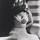I think what you’re getting at is solving simultaneous linear algebraic expressions.

ro_in_motion (2248)“Great Answer” (0) Flag as…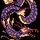I think you’re confusing symmetry with the commutative property… when you say “addition is symmetric.”

Though they can be related.

Symmetry has more to do with invariance. Something is symmetrical if, when transformed, it does not actually change. For example, if you flip around an equilateral triangle it looks the same, because it’s symmetrical. A square is symmetrical because it looks the same no matter which side is on “top.”

What you are talking about seems to have more to do with operations, specifically the order of operations. 2x + y + z is different from x + y + z because you have to do multiplication (2 times x) before you do addition (x+y+z). And, well, I’m not really sure how this is supposed to relate to symmetry. But I am not the brightest when it comes to math.

When you talk about generalized idea of symmetry, I think of Noether’s theorem. Some people say this is the most important idea in physics. It explains why some physical properties—for example, energy, or angular momentum—are conserved. It has to do with symmetry. No, I don’t actually understand it.

Qingu (21175)“Great Answer” (2) Flag as…I have heard about Noether’s Theorem and I am surprised that it is not better known. It really is mind blowing.

What I was trying to get at was that the equation could be any one of 2x + y + z or x + 2y + z or x + 2y + z, but which one applies will vary. This set of conditions is symmetric with respect to x, y and z. The definition of symmetric is that if you swapped x, y and z in any way, the conditions would remain the same.

LostInParadise (28461)“Great Answer” (0) Flag as…So what you’re talking about is the nature of the variable? Under what circumstances can you switch around variables?

I would say that 2x + y + z and x + 2y + z are only symmetrical (invariant) if you assume the designation of variables is arbitrary and can be switched. Which it basically is I guess, but then it’s just about semantics.

You could also say that 2 + 1 = 5 if you switched around the symbol for “1” and the symbol for “3,” because ultimately the symbols we use to designate quantity and variable are arbitrary.

Qingu (21175)“Great Answer” (0) Flag as…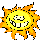I love it when you talk Math. I don’t pretend to understand, but it sounds so learned and neat.

Sunny2 (18812)“Great Answer” (0) Flag as…What don’t you understand? I can try to explain it. It’s pointless if you don’t understand what it means…

Qingu (21175)“Great Answer” (2) Flag as…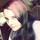Like an equilateral triangle, the letters x,y,and z can be swapped around and still have the same effect. But like an isosceles triangle, if it is set up like this: 2x+2y+z, the figure is not the same if you swap it around like this: x+2y+2z.
Or a scalene triangle: 2x+y+z to x+2y+z
That’s what ‘symmetrical’ means here.
I think it all depends on what type of triangle you’re working with.
My equilateral could go as follows: X=60 Y=60 Z=60
My first isosceles could go: X=30 Y=30 Z=60
My second isosceles could go:X=60 Y=30 Z=30
My first scalene could go:X=30 Y=60 Z=60
My second scalene could go: X=60 Y=30 Z=60
But they don’t add up to 180.
So one must be <60 for it to work.

BBawlight (2427)“Great Answer” (0) Flag as…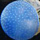The symmetry is in the Maths and Science because the Maths and Science are being used to describe things that have symmetrical properties.

dabbler (18354)“Great Answer” (0) Flag as…## Answer this questionor

#### Join

to answer.

This question is in the General Section. Responses must be helpful and on-topic.

Your answer will be saved while you login or join.
Have a question? Ask Fluther!
What do you know more about?
or
Knowledge Networking @ Fluther